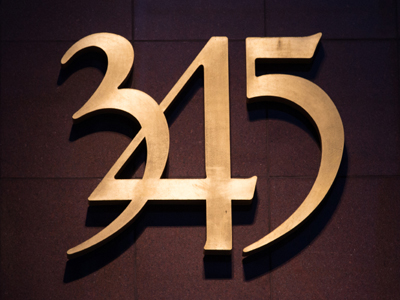345 is written three hundred and forty-five.

# Place Value (Year 3)

In KS2 Maths, kids will explore the value of numbers. Starting in Year Three, they'll understand that digits 0-9 have different values based on their position. They'll recognise ones, tens, and hundreds, and learn to write and convert numbers into words.

Place value involves hundreds, tens, ones, tenths, and hundredths. You've probably covered this in your maths lessons. For example, a 2 can mean 2, 20, or 200 depending on its place in a number. Take this quiz to test what you've learned about place value!

1.
What does the 8 represent in the number 804?
8
80
800
8,000
An 8 in the hundreds column = 8 x 100 which is 800
2.
When dealing with place value what is the name for the number immediately before the decimal point?
Tens
Hundreds
Ones
Thousands
Some people call the ones the units
3.
What does the 0 represent in the number 906?
0 tens
10
100
1,000
The zero in the ten column means there are no tens in the number but the zero still holds the ten's place
4.
What is this number in words - 407?
Four thousand and seven
Four hundred and seventy
Four hundred and seven
Forty seven
407 has 4 hundreds, 0 tens and 7 ones
5.
How many digits are there in our number system?
8
9
10
11
The ten digits are 0, 1, 2, 3, 4, 5, 6, 7, 8 and 9
6.
What does the 3 represent in the number 396?
3
30
300
3,000
As the three is in the hundreds column it represents 3 hundreds
7.
What is this number in figures - four hundred and seventy one?
4,071
400,701
4,710
471
471 has 4 hundreds, 7 tens and 1 one
8.
What is this number in figures - two hundred and one?
201
2,001
21
211
201 has 2 hundreds, 0 tens and 1 one
9.
What is this number in words - 359?
Three thousand, five hundred and nine
Three hundred and fifty nine
Thirty five hundred and nine
Three thousand and fifty nine
359 has 3 hundreds, 5 tens and 9 ones
10.
What does the 2 represent in the number 824?
2
20
200
2,000
A 2 in the tens column means 2 x 10 which is 20